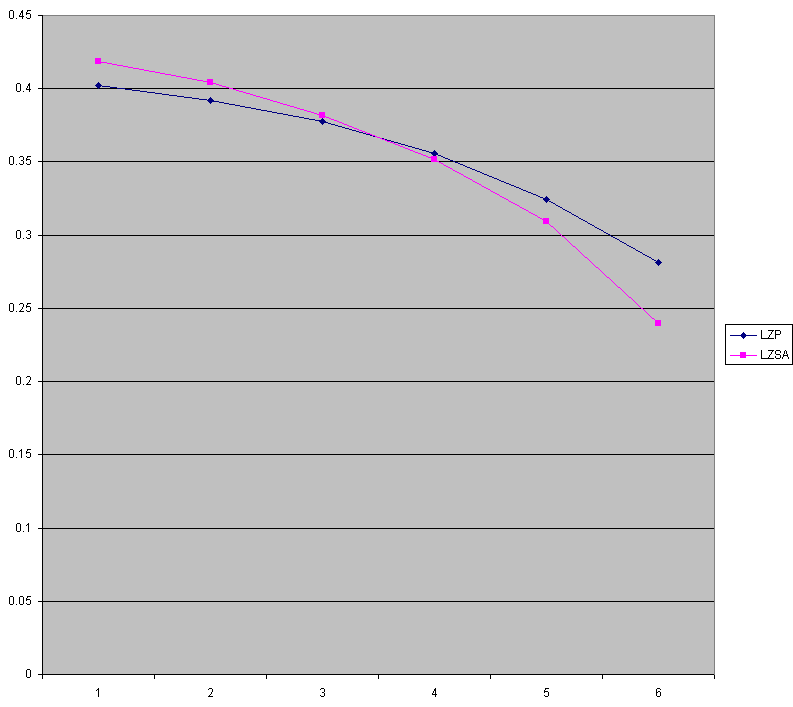# cbloom rants

## 2/09/2015

### 02-09-15 - LZSA - Some Results

So I re-ran some Oodle Network tests to generate some LZSA results so there's some concreteness to this series.

"Oodle Network" is a UDP packet compressor that works by training a model/dictionary on captured packets.

The shipping "OodleNetwork1 UDP" is a variant of LZP. "OodleStaticLZ" is LZSA-Basic and obviously HC is HC.

Testing on one capture with dictionaries from 2 - 64 MB :

```
test1   380,289,015 bytes

OodleNetwork1 UDP [1|17] : 1388.3 -> 568.4 average = 2.442:1 = 59.06% reduction
OodleNetwork1 UDP [2|18] : 1388.3 -> 558.8 average = 2.484:1 = 59.75% reduction
OodleNetwork1 UDP [4|19] : 1388.3 -> 544.3 average = 2.550:1 = 60.79% reduction
OodleNetwork1 UDP [8|20] : 1388.3 -> 524.0 average = 2.649:1 = 62.26% reduction
OodleNetwork1 UDP [16|21] : 1388.3 -> 493.7 average = 2.812:1 = 64.44% reduction
OodleNetwork1 UDP [32|22] : 1388.3 -> 450.4 average = 3.082:1 = 67.55% reduction
OodleNetwork1 UDP [64|23] : 1388.3 -> 390.9 average = 3.552:1 = 71.84% reduction

OodleStaticLZ  : 1388.3 -> 593.1 average = 2.341:1 = 57.28% reduction
OodleStaticLZ  : 1388.3 -> 575.2 average = 2.414:1 = 58.57% reduction
OodleStaticLZ  : 1388.3 -> 546.1 average = 2.542:1 = 60.66% reduction
OodleStaticLZ  : 1388.3 -> 506.9 average = 2.739:1 = 63.48% reduction
OodleStaticLZ  : 1388.3 -> 445.8 average = 3.114:1 = 67.89% reduction
OodleStaticLZ  : 1388.3 -> 347.8 average = 3.992:1 = 74.95% reduction

OodleStaticLZHC  : 1388.3 -> 581.6 average = 2.387:1 = 58.10% reduction
OodleStaticLZHC  : 1388.3 -> 561.4 average = 2.473:1 = 59.56% reduction
OodleStaticLZHC  : 1388.3 -> 529.9 average = 2.620:1 = 61.83% reduction
OodleStaticLZHC  : 1388.3 -> 488.6 average = 2.841:1 = 64.81% reduction
OodleStaticLZHC  : 1388.3 -> 429.4 average = 3.233:1 = 69.07% reduction
OodleStaticLZHC  : 1388.3 -> 332.9 average = 4.170:1 = 76.02% reduction

--------------

test2   423,029,291 bytes

OodleNetwork1 UDP [1|17] : 1406.4 -> 585.4 average = 2.402:1 = 58.37% reduction
OodleNetwork1 UDP [2|18] : 1406.4 -> 575.7 average = 2.443:1 = 59.06% reduction
OodleNetwork1 UDP [4|19] : 1406.4 -> 562.0 average = 2.503:1 = 60.04% reduction
OodleNetwork1 UDP [8|20] : 1406.4 -> 542.4 average = 2.593:1 = 61.44% reduction
OodleNetwork1 UDP [16|21] : 1406.4 -> 515.6 average = 2.728:1 = 63.34% reduction
OodleNetwork1 UDP [32|22] : 1406.4 -> 472.8 average = 2.975:1 = 66.38% reduction
OodleNetwork1 UDP [64|23] : 1406.4 -> 410.3 average = 3.428:1 = 70.83% reduction

OodleStaticLZ  : 1406.4 -> 611.6 average = 2.300:1 = 56.52% reduction
OodleStaticLZ  : 1406.4 -> 593.0 average = 2.372:1 = 57.83% reduction
OodleStaticLZ  : 1406.4 -> 568.2 average = 2.475:1 = 59.60% reduction
OodleStaticLZ  : 1406.4 -> 528.6 average = 2.661:1 = 62.42% reduction
OodleStaticLZ  : 1406.4 -> 471.1 average = 2.986:1 = 66.50% reduction
OodleStaticLZ  : 1406.4 -> 374.2 average = 3.758:1 = 73.39% reduction

OodleStaticLZHC  : 1406.4 -> 600.4 average = 2.342:1 = 57.31% reduction
OodleStaticLZHC  : 1406.4 -> 579.9 average = 2.425:1 = 58.77% reduction
OodleStaticLZHC  : 1406.4 -> 552.8 average = 2.544:1 = 60.70% reduction
OodleStaticLZHC  : 1406.4 -> 511.8 average = 2.748:1 = 63.61% reduction
OodleStaticLZHC  : 1406.4 -> 453.8 average = 3.099:1 = 67.73% reduction
OodleStaticLZHC  : 1406.4 -> 358.3 average = 3.925:1 = 74.52% reduction

```

Here's a plot of the compression on test1 ; LZP vs. LZSA-HC :Y axis is comp/raw and X axis is log2(dic mb)

What you should see is :

OodleNetwork1 (LZP) is better at small dictionary sizes. I think this is just because it's a lot more tweaked out; it's an actual shipping quality codec, whereas the LZSA implementation is pretty straightforward. Things like the way you context model, how literals & lengths are coded, etc. needs tweakage.

At around 8 MB LZSA catches up, and then as dictionary increases it rapidly passes LZP.

This is the cool thing about LZSA. You can just throw more data at the dictionary and it just gets better. With normal LZ77 encoding you have to worry about your offsets taking more bits. With LZ77 or LZP you have to make sure the data's not redundant or doesn't replace other more useful data. (OodleNetwork1 benefits from a rather careful and slow process of optimizing the dictionary for LZP so that it gets the most useful strings)

Memory use of LZSA is quite a bit higher per byte of dictionary, so it's not really a fair comparison in that sense. It's a comparison at equal dictionary size, not a comparison at equal memory use.